TitleCollege Algebra
Tutorial 26: Equations of LinesLearning Objectives

 After completing this tutorial, you should be able to: Know what  x- and y-intercepts are. Use the point/slope equation to set up an equation given any point on the line and the slope. Use the point/slope equation to set up an equation given two points on the line. Write a linear equation in the slope/intercept form.Introduction

 In this tutorial we will look closer at equations of straight lines. We will be going over how to come up with our own equations given certain information.  We will be using the slope of the line and a point it passes through to do this.  If you need a review on the slope of a line, feel free to go to Tutorial 25: Slope of a Line.  We will also be going over the concepts of x- and y-intercepts.  After you finish tutorials 25 - 28,  you will be an old pro at linear equations and graphing.  Let's see what we can do with x- and  y-intercepts and linear equations.Tutorial

 x-intercept

 The x-intercept is where the graph crosses the x axis. The word 'intercept' looks like the word 'intersect'.   Think of it as where the graph intersects the x-axis.  With that in mind,  what value is y always going to be on the x-intercept?  No matter where you are on the x-axis, y’s value is 0,  that is a constant.  We will use that bit of information to help us find the x-intercept.

 y-intercept

 If the x-intercept is where the graph crosses the x-axis where do you think the graph crosses for the y-intercept?  If you said the y-axis, you are absolutely right.  This time it is x’s value that is 0.  Anywhere you would cross the y-axis, x’s value is always 0.  We will use this tidbit to help us find the y-intercept.    Below is an illustration of a graph of a linear function which highlights the x and y intercepts: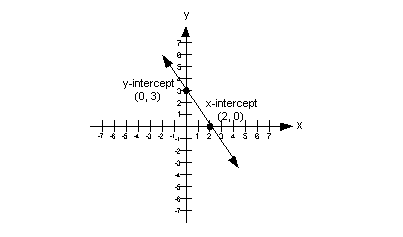In the above illustration, the x-intercept is the point (2, 0) and the y-intercept is the point (0, 3).   Keep in mind that the x- and y- intercepts are two separate points. There is only one point that can be both an x- and y- intercept at the same time, do you know what point that is? If you said the origin, (0, 0), give yourself a pat on the back.

 Point/Slope Form of an Equation A line going through the point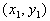and having slope of m would have the equation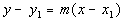We can use this form to plug into when we need to come up with a linear equation.  When writing an equation of a line, keep in mind that you ALWAYS need two pieces of information when you go to write an equation: ANY point on the line Slope Once you have these two pieces of information, you plug the x and y values from your point and the slope (m) value into the point/slope formula.

 Slope/Intercept Equation of a Line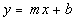In this form, m represents the slope and b represents the y-intercept of the line. Sometimes the directions will say to write the equation in the slope/intercept form.  Basically this means to solve the equation for y.  Note how y is by itself and everything else is on the other side.  Most times you will need to start the problem using the point/slope form and then you just solve for y to get it into the slope/intercept form.  Sometimes if you have it written in this form it makes it easier to work with when you graph.Example 1:  Write an equation for the line in point/slope form and slope/intercept form that has slope = -5 and passes through (2, 1).

 What are the two things we need to write an equation of a line???? If you said any point on the line and the slope, you are correct. Looks like we have all the information we need.  We are ready to put our equation together.  Point/Slope Form: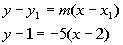*Point/slope form of the line

 Next, we want to write it in the Slope/Intercept Form, which basically means we need to solve for y: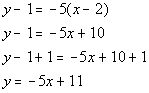*Dist. the -5 through (  ) *Inverse of sub. 1 is add 1 *Slope/intercept form of the line

 The equation of the line that passes through (2, 1) and has a slope of -5 is  y - 1 = -5(x - 2)    OR     y= -5x + 11.Example 2:  Write an equation for the line in point/slope form and slope/intercept form that has slope = 3 and passes through the origin.

 What are the two things we need to write an equation of a line???? If you said any point on the line and the slope, you are correct.   Do you know what the ordered pair for the origin is?  If you said (0, 0) you are right on!!!  That is the point that we will be using to plug into our equation. Looks like we have all the information we need.  We are ready to put our equation together.  Point/Slope Form: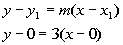*Point/slope form of the line

 Next, we want to write it in the Slope/Intercept Form, which basically means we need to solve for y:*Dist. the 3 through (  ) *Slope/intercept form of the line

 The equation of the line that passes through the origin and has a slope of 3 is  y - 0 = 3(x - 0)   OR   y = 3x.Example 3:  Write an equation for the line in point/slope form and slope/intercept form that passes through (-2, 1) and (2, 2).

 What are the two things we need to write an equation of a line???? If you said any point on the line and the slope, you are correct.   We have more than enough points. However, what about the slope?  Does this mean we can’t work out the problem?  You are not going to get off that easily.  We do have a way of finding the slope. Tutorial 25: The Slope of a Line shows us how we can get the slope given two points. Let’s find that slope: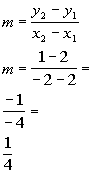*Slope formula *Plug in values  *Simplify

 OK, now we have our slope, which is 1/4.  Now it is just like examples 1 and 2 above, we want to put the slope and one point into the point/slope equation.  Note that you can use either point to plug in as long as it is a point that the line passes through.  I chose to plug in the point (-2, 1). Point/Slope Form: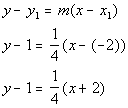*Point/slope form of the line

 Make sure that you are careful when one of your values is negative and you have to subtract it as we did in line 2.  x - (-2)  is not the same as x - 2.

 Next, we want to write it in the Slope/Intercept Form, which basically means we need to solve for y: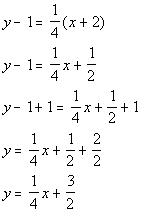*Dist. the 1/4 through (  ) *Inverse of sub. 1 is add 1       *Slope/intercept form of the line

 The equation of the line that passes through the points (-2, 1) and (2, 2) is  y - 1 = 1/4(x + 2)   OR   y = 1/4x + 3/2.Example 4:  Write an equation for the line in point/slope form and slope/intercept form that has an x-intercept of -2 and y-intercept of 1.

 What are the two things we need to write an equation of a line???? If you said any point on the line and the slope, you are correct. Do you know what the ordered pair is going to be for the x-intercept?  What about the y-intercept?  Above, we leaned that an x-intercept is where the line crosses the x-axis.  That means y’s value is always 0.  So the ordered pair for our x-intercept is (-2. 0). Above, we learned that an y-intercept is where the line crosses the y-axis.  That means x’s value is always 0.  So the ordered pair for our y-intercept is (0, 1).   We have more than enough points. However, what about the slope?  Does this mean we can’t work out the problem?  You are not going to get off that easily.  We do have a way of finding the slope. Tutorial 25: The Slope of a Line shows us how we can get the slope given two points. Let’s find that slope: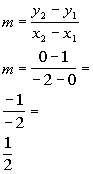*Slope formula *Plug in values  *Simplify

 OK, now we have our slope, which is 1/2.  Now it is just like examples 1 and 2 above, we want to put the slope and one point into the point/slope equation.  Note that you can use either point to plug in as long as it is a point that the line passes through.  I chose to plug in the point (-2, 0). Point/Slope Form: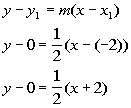*Point/slope form of the line

 Make sure that you are careful when one of your values is negative and you have to subtract it as we did in line 2.  x - (-2)  is not the same as x - 2.

 Next, we want to write it in the Slope/Intercept Form, which basically means we need to solve for y: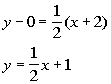*Dist. the 1/2 through (  ) *Slope/intercept form of the line

 The equation of the line that has an x-intercept of -2 and y-intercept of 1 is y - 0 = 1/2(x + 2)   OR   y = 1/2x + 1.Practice Problems

 These are practice problems to help bring you to the next level.  It will allow you to check and see if you have an understanding of these types of problems. Math works just like anything else, if you want to get good at it, then you need to practice it.  Even the best athletes and musicians had help along the way and lots of practice, practice, practice, to get good at their sport or instrument.  In fact there is no such thing as too much practice. To get the most out of these, you should work the problem out on your own and then check your answer by clicking on the link for the answer/discussion for that  problem.  At the link you will find the answer as well as any steps that went into finding that answer.Practice Problems 1a - 1c: Write an equation for the line in point/slope form and slope/intercept form that has the given condition.

 1a. Slope = ½ and passes through the origin. (answer/discussion to 1a)

 1b.  Passing through (-2, 4) and (-1, -1). (answer/discussion to 1b)

 1c. x-intercept = -5 and y-intercept = -2. (answer/discussion to 1c)Need Extra Help on these Topics?

The following are webpages that can assist you in the topics that were covered on this page:

 http://www.wtamu.edu/academic/anns/mps/math/mathlab/int_algebra/int_alg_tut16_eqline.htm This webpage helps you with writing equations of lines. http://www.purplemath.com/modules/strtlneq.htm This website helps you with writing linear equations.

Go to Get Help Outside the Classroom found in Tutorial 1: How to Succeed in a Math Class for some more suggestions.

Last revised on Feb. 6, 2010 by Kim Seward.